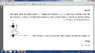# Angular momentum prob

• assaftolko
In summary, the system consists of two point masses, m and 2m, connected by a string of length L. At a certain moment, 2m is given a velocity of v0 in the positive x-axis direction. The system then starts to rotate about the center of mass due to conservation of angular momentum and the presence of a central force exerted by the tension in the string. The central force causes the system to rotate and the center of mass to move along the string. This can be understood through the principles of conservation of angular momentum and the principle of least action in Lagrangian mechanics.

#### assaftolko

Two point masses, with masses of m and 2m, are connected through a string that has a length of L. The two bodies are put on an horizontal frictionless table as in the figure, so that m is at the origin. The body 2m is above it (on the y axis) at a distance of L/2 m. At a certain moment they give 2m a velocity of v0 in the positive x-axis direction.

What's the kinetic energy of the system after the string is streched and m starts to move?

I realize that conservation of both linear and angular momentum applies here - but I don't understand something: Am I suppose to understand that the system starts to rotate about an axis which passes through the center of mass just as the string is streched? And if so - was there any "mathmatical way" for me to know the system starts rotating? Because it seems from the solution that when they calculated the angular momentum of the system as the string was streched - they used Icm*w to describe its angular momentum, while when they calculated the angular momentum of the system at the beginning (The picture phase) they used L=mvrsin(q) to describe the angular momentum as the sum of the angular momentum of 2m and m with respect to the center of mass...

#### Attachments

•Clipboard23.jpg
27.7 KB · Views: 314
Last edited:
was there any "mathmatical way" for me to know the system starts rotating?
Principle of least action. See "Lagrangian mechanics".

At certain moment in time the string will be fully extended. Work it out, and you will see that the center of mass will be on the string. From this point on, the string will be exerting a tensile force on both masses, and because the center of mass is on the string, in the center of mass frame (moving uniformly) you will have a central force, which means the masses are going to rotate about the center of mass. Well, the latter follows just as well from a generic application of conservation of angular momentum, but I hope the explanation above is somewhat more descriptive.

I don't understand what is this central force you are talking about..

The central force is the tension of the string.

If a system is rotating, there must an unbalanced force pointing at the center of rotation. "Central Force". In this case, as voko says, it comes from the tension in the string.

Another way of looking at it is to ask yourself, "What else could happen?" work out a few examples and see.

Simon Bridge said:
If a system is rotating, there must an unbalanced force pointing at the center of rotation. "Central Force". In this case, as voko says, it comes from the tension in the string.

Another way of looking at it is to ask yourself, "What else could happen?" work out a few examples and see.

Ok thanks a lot to you all!